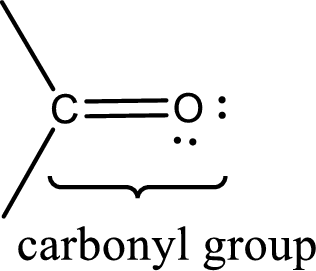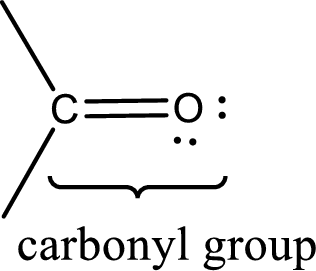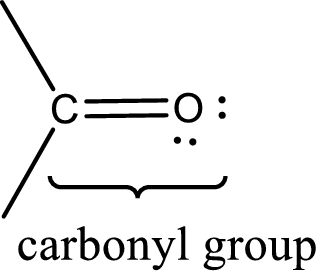Chapter 4, Problem 4.62EP### Organic And Biological Chemistry

7th Edition
STOKER + 1 other
ISBN: 9781305081079

#### Solutions

Chapter
Section### Organic And Biological Chemistry

7th Edition
STOKER + 1 other
ISBN: 9781305081079
Textbook Problem

# How many aldehyde groups and how many ketone groups are present in each of the following molecules? a. Progesterone b. 2-Heptanone c. Dihydroxyacetone d. Cinnamaldehyde

(a)

Interpretation Introduction

Interpretation:

The number of aldehyde groups and ketone groups present in progesterone has to be given.

Concept Introduction:

Carbonyl groups are the one which contain a double bond between carbon and oxygen atom.  Aldehydes and ketones possess this carbonyl functional group in it.  The structural representation of a carbonyl group can be given as shown below,The groups that is attached to the carbonyl carbon atom can be either hydrogen or carbon atom.  If the attached atoms are hydrogen and a carbon atom means then the compound is an aldehyde and if they are two carbon atoms means then the compound is a ketone.

Explanation

Carbonyl group contains a carbon atom and an oxygen atom.  The bond between carbon and oxygen in a carbonyl group is a double bond.

Structure of progesterone can be shown as given below,

(b)

Interpretation Introduction

Interpretation:

The number of aldehyde groups and ketone groups present in 2-heptanone has to be given.

Concept Introduction:

Carbonyl groups are the one which contain a double bond between carbon and oxygen atom.  Aldehydes and ketones possess this carbonyl functional group in it.  The structural representation of a carbonyl group can be given as shown below,The groups that is attached to the carbonyl carbon atom can be either hydrogen or carbon atom.  If the attached atoms are hydrogen and a carbon atom means then the compound is an aldehyde and if they are two carbon atoms means then the compound is a ketone.

(c)

Interpretation Introduction

Interpretation:

The number of aldehyde groups and ketone groups present in dihydroxyacetone has to be given.

Concept Introduction:

Carbonyl groups are the one which contain a double bond between carbon and oxygen atom.  Aldehydes and ketones possess this carbonyl functional group in it.  The structural representation of a carbonyl group can be given as shown below,The groups that is attached to the carbonyl carbon atom can be either hydrogen or carbon atom.  If the attached atoms are hydrogen and a carbon atom means then the compound is an aldehyde and if they are two carbon atoms means then the compound is a ketone.

(d)

Interpretation Introduction

Interpretation:

The number of aldehyde groups and ketone groups present in cinnamaldehyde has to be given.

Concept Introduction:

Carbonyl groups are the one which contain a double bond between carbon and oxygen atom.  Aldehydes and ketones possess this carbonyl functional group in it.  The structural representation of a carbonyl group can be given as shown below,The groups that is attached to the carbonyl carbon atom can be either hydrogen or carbon atom.  If the attached atoms are hydrogen and a carbon atom means then the compound is an aldehyde and if they are two carbon atoms means then the compound is a ketone.

### Still sussing out bartleby?

Check out a sample textbook solution.

See a sample solution

#### The Solution to Your Study Problems

Bartleby provides explanations to thousands of textbook problems written by our experts, many with advanced degrees!

Get Started